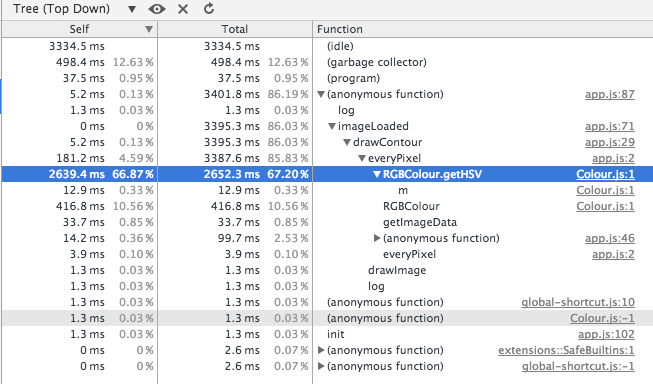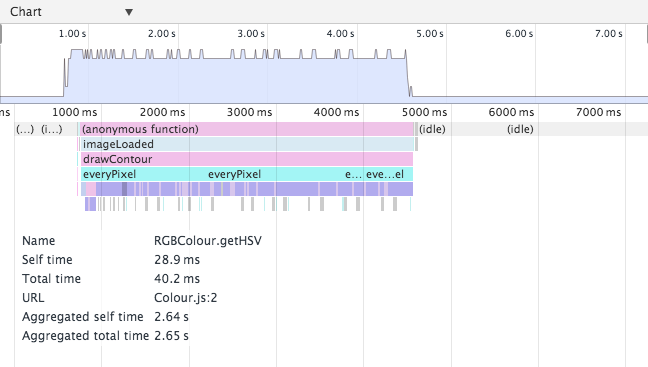# JavaScript pixel processing

Problem: thresholding an image using a HTML5 2D canvas. It’s a really simple problem, but if implemented wrong it can lead to really bad performance.

My initial idea was to make a function everyPixel(ctx, f) that applies f to every pixel of ctx. And then f would deal with all the pixel processing and drawing.

Basic implementation:

function everyPixel(ctx, f) {
var width = ctx.canvas.width;
var height = ctx.canvas.height;

for (var i=0;i<width;i++) {
for (var j=0;j<height;j++) {
var col = ctx.getImageData(i,j,1,1).data;
f(col, {x:i, y:j});
}
}
}


And the thresholding I was trying to apply (includes Colour.js for colour space transformation):

everyPixel(imgctx, function(colour, pos) {
var col = new RGBColour(colour, colour, colour, colour);
var hsv = col.getHSV();
if (hsv.v < threshold) {
// make black
imgctx.fillRect(pos.x, pos.y, 1, 1);
} else {
// clear
imgctx.clearRect(pos.x, pos.y, 1, 1);
}
});


But this is really slow, because

1. it gets the image data pixel by pixel,
2. it uses fillRect and clearRect to draw, which is slower than directly using putImageData, and
3. it does that for every single pixel instead of as a batch operation.

So my function needs some changes to address these two performance issues.

Changing the everyPixel function to get all the image data at once, iterate over all pixels in the data, and then redraw the obtained image data results in a faster method. This is the updated code:

function everyPixel(ctx, f) {
var imageData = ctx.getImageData(0, 0, ctx.canvas.width, ctx.canvas.height);
var data = imageData.data;

for (var i=0;i<data.length;i+=4) {
f(data, i);
}

ctx.putImageData(imageData, 0, 0);
}


Now f does not take x,y coordinates anymore, but it takes the index at which the processing starts and the data array. Working out the coordinates of the current pixel given the canvas size and pixel index is trivial and left as an exercise for the reader.

ImageData objects contain an array of pixel values, four integer values in the interval [0-255] (inclusive) per pixel in the RGBA order (see on MDN). Accessing our pixel values within f(data, index) is as simple as

data[index]   // R
data[index+1] // G
data[index+2] // B
data[index+3] // A


And here goes our new f function to apply the thresholding:

everyPixel(imgctx, function(data, index) {
var col = new RGBColour(
data[index],
data[index+1],
data[index+2],
data[index+3]);

var hsv = col.getHSV();

data[index] = 0;
data[index+1] = 0;
data[index+2] = 0;

if (hsv.v < threshold) {
// make black (full opacity)
data[index+3] = 255;
} else {
// make transparent
data[index+3] = 0;
}
});


On my Macbook Air, on Chrome, it finished in around 3400ms to 3500ms. Massive improvement from before (don’t have times, but it was slow enough that I didn’t wait for it to finish). However, still unacceptably slow. Let’s measure what’s going so terribly bad.

A simple profiling in Chrome reveals that Colour.js is quite slow. 67% of the time is spent converting between colour spaces, as it can be observed in the screenshots above.Here comes the question, can I apply the same thresholding on RGBA directly? Likely yes. Changing the function to the following does the trick for me. New code:

everyPixel(imgctx, function(data, index) {
// my image is not transparent initially,
// so not filtering on alpha at all.
if (data[index]   < threshold &&
data[index+1] < threshold &&
data[index+2] < threshold) {

// make black
data[index] = 0;
data[index+1] = 0;
data[index+2] = 0;
data[index+3] = 255;
} else {
// make transparent
data[index] = 0;
data[index+1] = 0;
data[index+2] = 0;
data[index+3] = 0;
}
}


Update: The code above does exactly the same as the thresholding I was applying on the HSV colour space, because v = max(r,g,b) (source). Thanks Marius for the observation and link.

Now this is good enough. The operation now takes 20-30 ms.

Out of curiosity, I tried the initial version without Colour.js. It was certainly faster than the Colour.js version but still too slow for me to let it finish.

I added all the code on a JSFiddle at https://jsfiddle.net/0vuf78t1/.